Students can access the CBSE Sample Papers for Class 12 Chemistry with Solutions and marking scheme Term 2 Set 6 will help students in understanding the difficulty level of the exam.

## CBSE Sample Papers for Class 12 Chemistry Term 2 Set 6 with Solutions

Time Allowed: 2 Hours
Maximum Marks: 35

General Instructions:

• There are 12 questions in this question paper with internal choice.
• SECTION A – Q. No. 1 to 3 are very short answer questions carrying 2 marks each.
• SECTION B – Q. No. 4 to 11 are short answer questions carrying 3 marks each.
• SECTION C- Q. No. 12 is case based question carrying 5 marks.
• All questions are compulsory.
• Use of log tables and calculators is not allowed.

Section – A
(Section A-Question No 1 to 3 are very short answer questions carrying 2 marks each.)

Question 1.
Name the reagents used in the following reactions: (Any two) (2)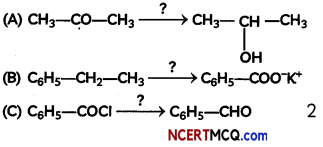(A)
LiAlH4

Explanation:(B) KMnO4/KOH, heat

Explanation:
When ethyl benzene is oxidised with alkaline KMnO4 or acidic Na2Cr2O7, the entire side chain (in benzene homologues) with at least one H at a carbon, regardless of length is oxidised to -COOH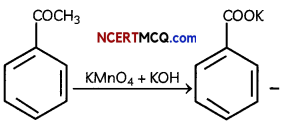(C) H2/Pd – BaSO4

Explanation:
Rosenmund reduction is a hydrogenation process in which an acyl chloride is selectively reduced to an aldehyde. The reaction was named after Karl Wilhelm Rosenmund who first reported it.

So, benzoyl chloride is reduced to benzaldehyde in presence of Pd-BaSO4 and H2.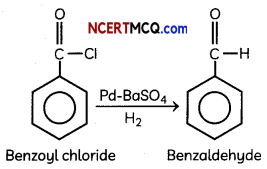Question 2.
Give reason
(A) Boiling point of methyl amine is 60°C while that of trimethyl amine is 30°c. State reason for this difference in boiling point.
Trimethyl amine is a tertiary amines and it does not have hydrogen atoms bonded to the nitrogen atom and therefore are not hydrogen bond donors. Thus tertiary amines cannot form intermolecular hydrogen bonds. As a result., they have lower boiling points than primary amine like methyl amine.

(B) Why does Aniline not react with dilNaOH? (2)
Aniline does not react with dil. NaOH because in basic conditions, the molecule is uncharged and only very slightly polar. Its polarity is so low that interactions between water and the NH2 group are not sufficient to make the molecule, souble in water.Question 3.
Identify which of the following AX, AY or AZ indicates a strong .weak or a non electrolyte. (2)AX – fig (a) indicates a non electrolyte as the particles do not dissociate at all.
Examples:

AY -fig (b) indicates a Weak Electrolytes: as the particles are not completely dissociated into ions in solution.
Examples: CH3COOH, NH4OH, HCN etc.

AZ -fig (c) indicates a Strong Electrolytes: In these electrolytes particles completely dissociateinto ions in solution.
Examples: HCl, KCl, NaOH, NaCl

Section – B
(Section B-Question No 4 to 11 are short answer questions carrying 3 marks each.)

Question 4.
(A) Identify the Coordination complex which contain Cyanide as the ligand and Iron as the central metal atom. This complex is used to identify both Cu2+ and Fe3+ cation during salt analysis.
The coordination complex is K4[Fe(CN)6]

Related Theory:
Both Cu2+ and Fe3+ are detected with potassium fer- rocyanide in the following manner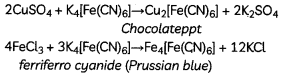(B) While NH2 is a strong ligand but NH4+ is not. Explain.
Ammonia is converted into ammonium ion in the following manner:
NH3 + H+ ⇌ NH4+

Complexes are formed by donation of a pair of electrons from ligand to metal. In ammonia, N atom has one lone pair of electrons. So it can form complexes. Nitrogen donates this lone pair of electrons to proton to form ammonium ion. NH4+ ion does not possess any lone pair of electrons which it can donate to central metal ion hence it does not form complexes.

(C) Select bidentate of didentate ligand from the following? (3)
CO, SCN, CH3COO, C2O42-
Bidentate Ligand are the one which donates 2 pair of electrons to metal atom. Among the options provided, C2C>42_ is the only Ligand which is bidentate ligand. It donates electrons from its 2 oxygen atoms.

Question 5.
(A) Bleeding due to a small cut is stopped by applying alum.
Alum is used to stop blood from a cut. It allows the blood clot to form. The high ionic strength promotes flocculation of the blood . Thus, blood is positive sol and sol particles are coagulated more efficiently by S042~ ions

(B) You must have observed at sunset an orange colour develops in the sky .Why is it so?
At the time of sunset, the sun is at horizontal. The Light emitted by the sun has to travel a relatively longer distance through the atmosphere. As a result, blue part of light is scattered away by the particulate (colloidal particles)in the atmosphere causing red part to be visible.

(C) Why is Chemisorption called activated absorption? (3)
Chemisorption is also called as activated adsorption because there is an involvement of chemical bond formation between the reactants and the adsorbent. This formation of chemical bonds requires a high activation energy.

Related Theory:
Chemisorption is highly specific in nature as the molecules are held on the solid surface through a chemical bond. For eg., oxygen is adsorbed on metals due to oxide formation and also hydrogen gets adsorbed on metals due to hydride formationQuestion 6.
(A) Name the cell that drives a non-spontaneous redox reaction through the application of electrical energy with the help of a suitable example
An electrolytic cell is an electrochemical cell that drives a nonspontaneous redox reaction through the application of electrical energy.

Example: The decomposition of water into hydrogen and oxygen, and bauxite into aluminum and other chemicals.

(B) How much electricity in Faraday is required to produce
(i) 80g of Ca from molten CaCl2?
From given data,
Ca2+ + 2e → Ca

Electricity required to produce 40 g of calcium = 2 F
Therefore, electricity required to produce
80 g of calcium = $$\frac{2 \times 80}{40}$$F
= 4 F

(ii) 54g of Al from molten Al2O3?
OR
A voltaic cell is set up at 25°C with the following half cells:
$$\frac{\mathrm{Al}}{\mathrm{Al}^{3+}}$$ (0.001M) and $$\frac{\mathrm{Ni}}{\mathrm{Ni}^{2+}}$$ (0.50M).
Write an equation for the reaction that occurs when the cell generates an electric current and determine the cell potential. (3)
From given data
Al3+ + 3e → Al

Electricity required to produce 27 g of Al
= 3 F

Therefore, electricity required to produce 54g of Al = $$\frac{3 \times 54}{27}$$F
= 6 F
OR
E0(Al3+/Al) = – 1.66V, E0(Ni2+/Ni) = -0.25V

Anode: [Al → Al3+ + 3e] × 2
Cathode: [Ni2+ + 2e → Ni] × 3

Net cell reaction: 2Al +3Ni2+ → 2Al3++ 3Ni
n = 6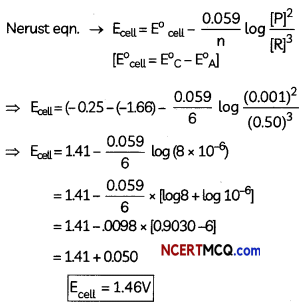Caution:
Students should not forget to put powers of stoichiometric coefficients on the concentrations of products and reactants in Nernst equation.

Question 7.
Account for the following:
(A) Which metal in the first transition series (3d series) exhibits +1 oxidation state most frequency and why?
Copper exhibits + 1 oxidation state more frequently i.e., Cu+1 because of its electronic configuration 3d104s1. It can easily lose 4s1 electron to give stable 3d10 configuration.

(B) Which of the following cations are coloured in aqueous solutions and why? SC3+, V3+, Ti4+, Mn2+.
(At. nos. Sc = 21, V = 23, Ti = 22, Mn = 25)
Sc3+ = 4S° 3d3 = no unpaired electron
V3+ = 3d2 4s° = 2 unpaired electron
Ti4+ = 3d° 4s° = no unpaired electron
Mn2+ = 3d5 4s° = 5 unpaired electron
Thus V3+ and Mn2+ are coloured in their aqueous solution due to presence of unpaired electron.

(C) The transition metals (with the exception of Zn, Cd and Hg) are hard and have high melting and boiling points. In Zn, Cd and Hg, all the electrons in d-subshell are paired. Hence, the metallic bonds present in them are weak (3)
The transition metals (with the exception of Zn, Cd and Hg) are hard and have high melting and boiling points because of stronger metallic bonding and high enthalpies of atomization.

Question 8.
(A) Give the product for: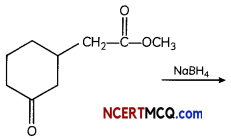NaBH4 does not reduce ester group. It is mild reducing reagent which reduces aldehyde and ketone into their respective alcohols.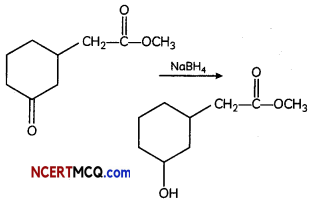(B) Which among the following will undergo cannizaro reaction? Benzaldehyde, acetone .formaldehyde
Benzaldehyde and formaldehyde undergo cannizaro reaction as these compounds do not contain alpha hydrogen

Related Theory:
An alpha carbon is the first carbon that is joined to the functional group. In the case of aldehydes and ketones, a functional group is a carbonyl group. The functional group is responsible for the formation of alpha hydrogen.(C) Iodoform reaction is obtained when methyl ketones react with hypoiodite not with iodine. Why? (3)
Hypoiodite ion is very strong oxidising agent .hence it can oxidise methyl ketone to iodoform, iodide ion is a reducing agent and hence it cannot oxidise methyl ketone to iodoform.

Related Theory:
Reaction of Iodine with NaOH gives sodium hypoiodite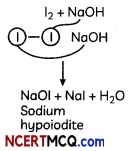The Haloform Reaction;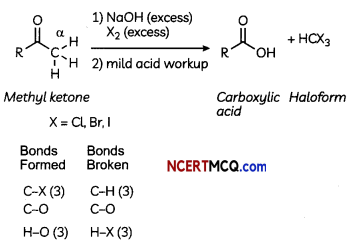Formation of carboxylic acids only happens with methyl ketones
Iodoform test (NaOH/I2) is historically valuable for identifying methyl ketones

Question 9.
Write the IUPAC names of the foLlowing coordination compounds
(A) [Cr(NH3)3Cl3]
(B) K4[Fe(CN)6]
(C) [CoBr2(en)2]+, (en = ethylenediamine)
OR
(A) Write the formulae for the following coordination compounds:
(i) Tetraammineaquachloridocobalt (III) chloride
(ii) Potassium tetracyanonickelate (II)
(B) Write the hybridization of the complex
[NiCl4]2-. (Atomic number of Ni = 28) (3)
(A) Triamminetrichloridochromium (III)

(B) Potassiumhexacyanidooferrate (III)

(C) Dibromidobis (ethane 1, 2-diamine) cobalt (III)
OR
(A) (i) [Co(NH3)4(H2O)Cl]Cl2
(ii) K2[Ni(CN)4]

(B) Ni(28) : [Ar] 4s23d8
Ni2+: [Ar] 4s°3d8
Since Cl is a weak field ligand, the pairing of electrons will not take place.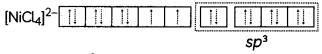It has sp3 hybridization. It has a tetrahedral shape and complex is paramagnetic in nature due to presence of unpaired electrons.

Question 10.
Write the structures of the main product when acetone (CH3—CO—CH3) reacts with the following reagents.
(A) Zn—Hg/Conc. HCl
(B) H2N—NHCONH2/H+
(C) CH3MgBr and then H3O+
OR
An organic compound (A) (molecular formula C8H16O2) was hydrolysed with dilute sulphuric acid to give a carboxylic acid (B) and an alcohol (C). Oxidation of (C) with chromic acid also produced (B). On dehydration (C) gives but-l-ene. Write the equations for the reactions involved. (3)Related Theory:
Clemmensen reduction is used to reduce aldehydes and ketones to hydrocarbons. Zinc amalgam and concentrated hydrochloric acid are used as Clemmensen’s reagent Clemmensen reduction is a reaction which involves the reduction of a carbonyl compound to form a simple hydrocarbon.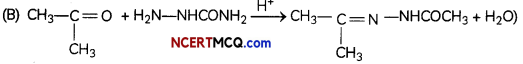Related Theory:
Semicarbazones are derivatives of aldehydes and ketones produced by the condensation reaction between a ketone or aldehyde and semicarbazide. Semicarbazones are useful for identification and characterization of aldehydes and ketones.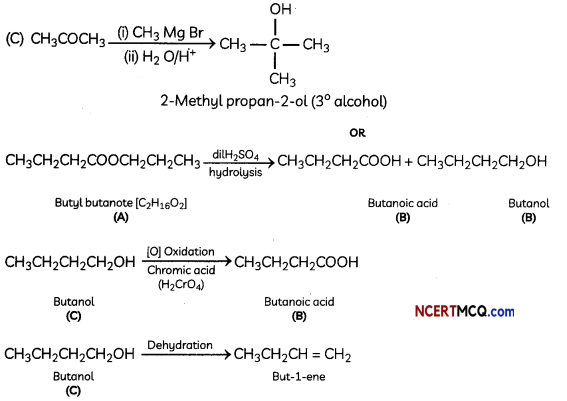Explanation:
Organic compound A is an ester as on acid hydrolysis it gives a mixture of an acid and an alcohol.
Oxidation of alcohol (C) gives acid (B). Hence, the number of carbon atoms in (B) and (C) are same.
Ester (compound A) has eight C atoms. Hence, both carboxylic acid B and alcohol C must contain 4 C atoms each.
Dehydration of alcohol C gives but-l-ene. Hence, C must be a straight chain alcohol, i.e butan-l-ol Oxidation of (C) gives (B). Hence, (B) is butanoic acid.

Question 11.
Write the chemical equations involved when aniline is treated with the following reagents:
(i) Br2 water
(ii) CHCl3 + KOH
(iii) HCl
OR
Explain giving suitable reason:
(i) Aniline does not undergo Friedel-Crafts reaction.
(ii) p-methylaniline is more basic than p nitroaniline.OR
(i) Since aniline is basic, it reacts with Lewis acid AlCl3 to form salt. .
(ii) It is because methyl group in p methylaniline is electron releasing, it donates electrons for donation whereas nitrogroup in p nitroaniline is electron withdrawing.
(iii) Since -NH2 group is ortho -para directing hence acetylation is done so as to decrease the reactivity of Aniline towards electrophilic substitution reactions.Section – C
(Section C-Question No 12 is case-based question carrying 5 marks.)

Question 12.
Reaction 1 Initiation
CH3CHO → CH3 + CHO
Propagation
CH3 + CH3CHO → CH3CO + CH4
CH3CO → CH3 + CO

Termination
2 .CH3 → C2H6

Reaction 2
decomposition of acetaldehyde is an example of fractional order reaction because the order of the reaction is a fractional value.
It can be a whole number or a fraction. It can be noted that when the order of reaction is a fraction, the reaction is generally a chain reaction or follows some other complex mechanism. An example of a chemical reaction with a fractional reaction order is the pyrolysis of acetaldehyde.

The rate of decomposition of acetaldehyde into methane and CO in the presence of 12 at 800 K follows the rate law:
Rate = k[CH3CHO][I2l
The decomposition is believed to go by a two- step mechanism:
CH3CHO + I2 → CH3I + CO + HI
CH3I + HI → CH4 + I2
(A) Which of the two steps is a slower one?
(B) Explain why order cannot be determined by a balanced chemical equation
(C) Give one example of fractional order reaction.
(D) A reaction is of first order in reactant A and of second order in reactant B. How is the rate of this reaction affected when:
(i) the concentration of B alone is increased to three times.
(ii) the concentrations of A as well as B are doubled?
OR
A reaction is of second order with respect to a reactant. How is the rate of reaction affected if the concentration of the reactant is reduced to half? What is the unit of rate constant for such a reaction? (5)
(A) Rate law is obtained from the slowest step Hence step 1: CH3CHO + I2 → CH3I + CO + HI this step is the slower one Here I2 is the catalyst as it is consumed in first step and regenerated in the next step.

(B) The sum of powers of the concentration of the reactants in the rate law expression is .called the order of that chemical reaction. Order cannot be determined by a balanced chemical equation it may or may not be equal to the sum of stoichiometric coefficients as it is an experimental property.

(C) Decomposition of acetaldehyde Explanation- The order of the decomposition of acetaldehyde is 3/2 or 1.5.

(D) r = k[A]1 [B]2
(i) When concentration of B increases to 3 times, the rate of reaction becomes 9 times
r = kA(3B)2 ∴ r = 9kAB2 = 9 times

(ii) r = k(2A) (2B)2 ∴ r = 8kAB2 = 8 times
OR
Rate = k [A]2 = ka2
If [A] = $$\frac{1}{2 a}$$; Rate = k $$\left(\frac{a}{2}\right)^{2}=\frac{1}{4}$$ka2

∴ Rate = 1/4th (one fourth of original rate)
The unit of rate constant is L mol-1 s-1